# Homework 3 Sand

For this project you will write 2-d algorithmic code to implement a kind of 2-d world of sand. What my kids have described as the world's worst version of Minecraft. When it's working, it's kind of fun to play and watch in its low-key way.

The starter code handles setting up the GUI window, and handling the controls and drawing. All the logic that makes the world work will be built by you.

Homework 3 is due Wed Oct 13th at 11:55 pm. To get started, download sand.zip.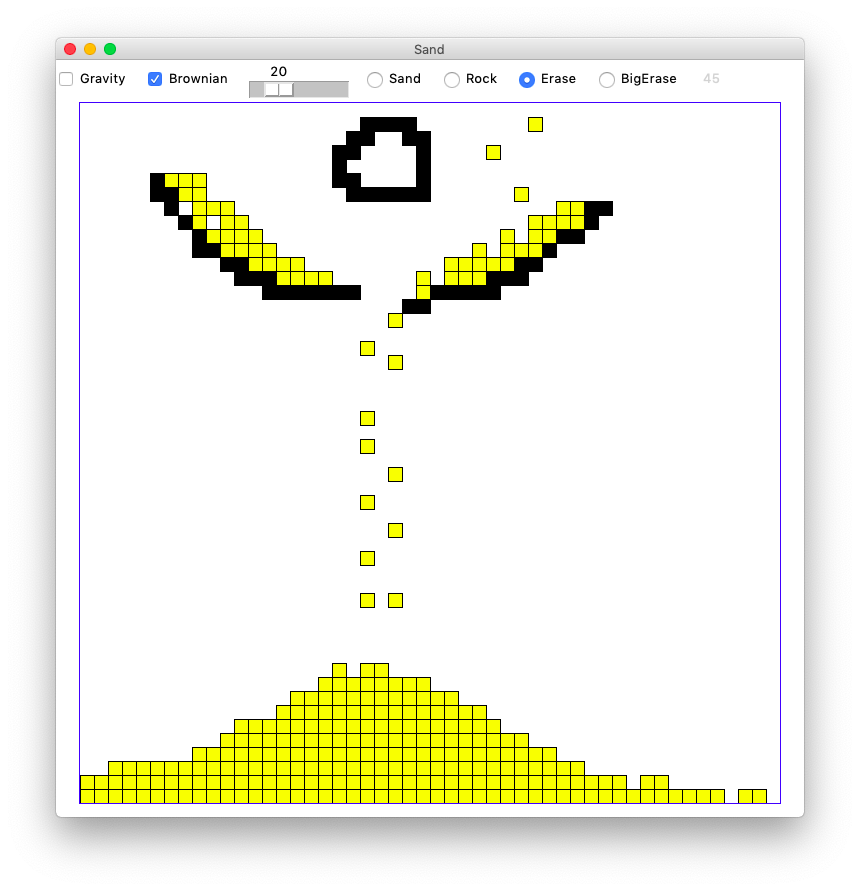## Grid Class

We will use the simple CS106A Grid utility class to store the 2-d date. See Grid Reference

Using the Grid looks like this

```grid = Grid(3, 2)      # make 3 by 2 grid, initially all None
grid.set(0, 0, 'hi')   # set a value at 0,0
val = grid.get(0, 0)   # get a value out at 0,0

if grid.in_bounds(4, 5):  # is 4,5 in bounds?
...
```

## Warmup - Waterfall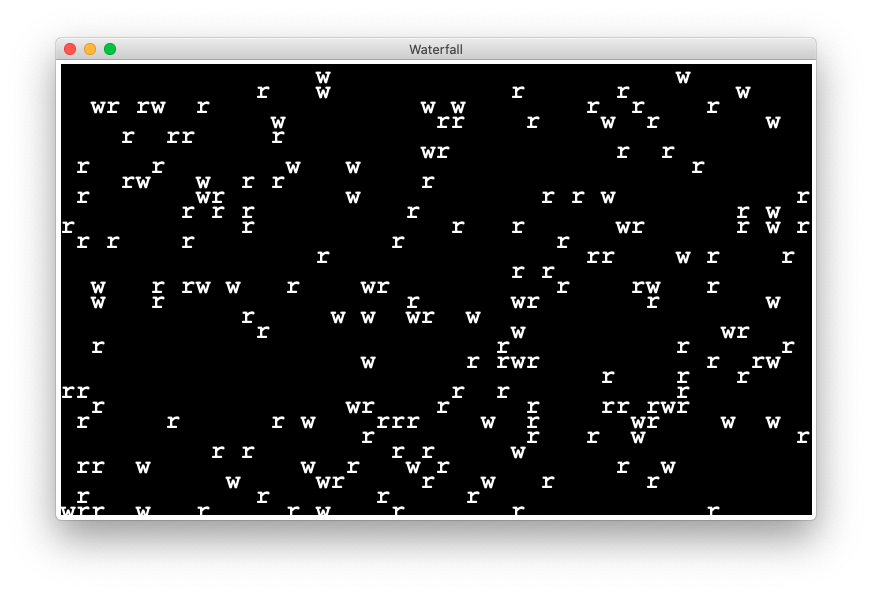See the file waterfall.py - there is one function for you to write in here as a warmup.

In the waterfall grid every square is either rock `'r'`, water `'w'`, or empty `None`.

The waterfall world is a little more simple than the sand world. Every turn, every water moves. The only question is, which way will the water move, or will it disappear from the world.

## down_dx()

The down_dx() function takes in the x,y of a square of water in the world, and returns its "dx" indicating which way that water will move. There are no loops in this function. It works on a single x,y. Note: don't check if there is water is at x,y just assume it is there, since that is the official precondition for down_dx(). The function that calls down_dx() does the needed checking for the water, and actually erases it in preparation for the move.

There are four possible values of dx. Check for the four cases in the order below, returning the first option that works.

0 - If the square directly below the water is in-bounds and empty, the water will move there. (return 0)

-1 - If the square down-left is in-bounds and empty, the water will move there (return -1)

1 - If the square down-right is in-bounds and empty, the water will move there (return 1)

`None` - a dx of None signals that none of the 3 moves 0/-1/1 is available - they are all either out of bounds or non-empty, so the water should disappear. (return `None`)Why are there no loops in down_dx()? Because the x,y square to work on is specified in the passed-in parameter values, e.g. x=3 y=4 means look at that square, and no loops are required to accomplish that. Where are the x,y loops then? The x,y loops are in the do_gravity() function which loops over the whole world, calling down_dx() from inside the loops to hit every x,y.

## down_dx() Code

Write code for the down_dx() function in waterfall.py. First check for the straight down path (dx = 0). If that works return 0. Otherwise try down-left, returning -1 if that works, and so on. The `return None` should be the last line, running only if none of the other options panned out.

Doctests are provided for down_dx(). Run the Doctests to work out your down_dx() code as you go.

Here are the down_dx() Doctets:

```    >>> grid = Grid.build([[None, 'w', 'w', 'w', 'w', None], [None, None, 'r', 'r', 'w', None]])
>>> down_dx(grid, 1, 0)  # try 'w' at 1,0
0
>>> down_dx(grid, 2, 0)  # try 'w' at 2,0
-1
>>> down_dx(grid, 3, 0)  # blocked (">>>" below means None result)
>>>
>>> down_dx(grid, 4, 0)
1
>>> down_dx(grid, 4, 1)  # blocked by out of bounds
>>>
```

Here is a visualization of the 6 by 2 grid used for the Doctests:

```    0     1     2    3    4    5
0 [None, 'w',  'w', 'w', 'w', None]
1 [None, None, 'r', 'r', 'w', None]
```

A quirk of the Doctest syntax is that a function result of `None` is encoded in the Doctest by writing literally nothing before the next ">>>" - this is shown in the 3rd and 5th calls to down_dx() above.

## Look at move_water()

The code for this function is provided. Look it over. It is called once for every water in the world. It calls your down_dx() function to see which way the water should go. If dx is `None`, the water is erased. Otherwise it is moved to `x + dx` horizontally and `y + 1` vertically (i.e. down). There are no tests for this function.

## Run From Command Line

Once your down_dx() passes all its Doctests, run the waterfall program from the command line. If you look carefully, you should see the three cases. Rain dodging to the left around rocks is common, and in rare cases water going to the right when hitting the right of two rocks, and very rarely rain disappearing when hitting the middle of three rocks. You may need to run a few times to get a random world that exhibits all the cases.

```\$ python3 waterfall.py
```

Of course watching the program run in realtime, it's impossible to see that it's behaving correctly all the time. We use the small, frozen-in-time Doctests to really see (and debug!) code like this.

## The Sand Program

Sand is a bigger program, and we want the advantages of a divide-and-conquer strategy. We'll build the program up as a series of decomposed functions. We will leverage Python Doctests, testing each function in isolation before moving on to the larger functions. You need to write 5 functions to make the world work.

## How Does The Sand Grid Work?

Every square in the Sand grid holds one of three things:

1. Sand represented by `'s'`

2. Rock represented by `'r'`

3. Empty represented by `None`

Here is a 3 by 2 grid with some rocks and one sand. The 's' is at x=1 y=0 (aka 1,0) with our usual origin upper-left system.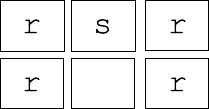If we move the sand down from 1,0 to 1,1 the grid looks like this: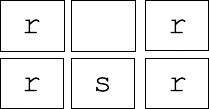Moving sand down by one square like this is the central operation of this game.

## a. do_move()

The code for do_move() is provided. It's only two lines long. The """Pydoc""" defines what this function needs to do: move a sand from x_from,y_from to x_to,y_to, and return the changed grid to the caller. The function assumes that the move is legal (different code checks that). Two Doctests are provided.

```def do_move(grid, x_from, y_from, x_to, y_to):
"""
Given grid and x_from,y_from with a sand,
and x_to,y_to. Move the sand to x_to,y_to
and return the resulting grid.
Assume that this is a legal move: all coordinates are in
bounds, and x_to,y_to is empty.
(i.e. a different function checks that this is a
legal move before do_move() is called)
(provided code)
>>> grid = Grid.build([['r', 's', 's'], [None, None, None]])
>>> do_move(grid, 1, 0, 1, 1)
[['r', None, 's'], [None, 's', None]]
>>>
>>> grid = Grid.build([['r', 's', 's'], [None, None, None]])
>>> do_move(grid, 2, 0, 2, 1)
[['r', 's', None], [None, None, 's']]
"""
```

The code for do_move() is short, but it is good example of using Doctests to spell out test cases. Here is the first Doctest which moves the 's' at 1,0 down to 1,1.

```    >>> grid = Grid.build([['r', 's', 's'], [None, None, None]])
>>> do_move(grid, 1, 0, 1, 1)
[['r', None, 's'], [None, 's', None]]
```

What the 3 lines of the Doctest do:

1. `grid = ..` This sets up a grid variable for the next 2 lines. The grid is tiny: width 3 height 2, just enough to write a little test.

2. `do_move(grid, 1, 0, 1, 1)` - calls the do_move() function, moving the 's' at 1,0 to 1,1. The function returns the changed grid.

3. The third line shows what the grid should look like post-move: `[['r', None, 's'], [None, 's', None]]`. The Doctest machinery verifies that the grid coming out of do_move() matches this written state.

Run the Doctests and they should pass. Doctest pro-tip: when you have run a Doctest once, you can re-run it by clicking the green "play" button at the left-edge of the window. Running a Doctest again and again is the common pattern as you work on the functions below.

## b. is_move_ok() Rules

The is_move_ok() function is given a prospective x_from,y_from and x_to,y_to for a move, and returns True if the move is ok, or False otherwise. The grid is not changed by this operation. Much of the complexity in this whole program is in this function, so we will give it a thorough testing.

Here is the Pydoc for the is_move_ok() function.

```def is_move_ok(grid, x_from, y_from, x_to, y_to):
"""
Given grid and x_from,y_from and destination x_to,y_to.
Assume there is sand at x_from,y_from. Is it possible
to move to x_to,y_to?
The from location is always in bounds, but the to location
may not be. Return True if the move is ok, or False otherwise.
Ok move: to location is in bounds, empty, not violating corner rule.
"""
```

We'll call x_to,y_to the "destination" of the move. In the Sand world, there are five possible moves: left, right, down, down-left, and down-right. Here are the rules for a legal move:

### 1. OOB Rule

If the destination is out of bounds of the grid (OOB), the move is not ok.

Three Doctests for this rule are provided. These tests build a 1 row by 1 col grid, checking that a few out-of-bounds moves return False.

```    >>> # Provided out-of-bounds tests
>>> # Make a 1 by 1 grid with an 's' in it to check in-bounds cases
>>> grid = Grid.build([['s']])
>>> is_move_ok(grid, 0, 0, -1, 0) # left blocked
False
>>> is_move_ok(grid, 0, 0, 0, 1)  # down blocked
False
>>> is_move_ok(grid, 0, 0, 1, 1)  # down-right blocked
False
```

### 2. Empty Rule

The destination square in the grid must be empty, or the move is not ok.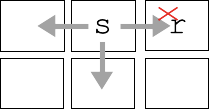Above is a picture os a single 's' at 1,0 in a 3 by 2 grid. The move left to 0,0 and the move down to 1,1 are both ok (return True). The move right to 2,0 is bad, blocked by the the 'r' there (return False).

### 3. Corner Rule

For a diagonal down-left or down-right move, the corner square must be empty (None).

Consider the down-left and down-right moves of the 's' here: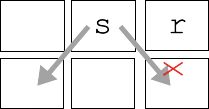The corner rule: for a down-left or down-right move, the corner square above the destination must be empty. So in the picture, the down-left is ok since 0,0 is empty, but down-right is bad due to the rock at 2,0. Sand at 2,0 would also block the move.

## is_move_ok() Doctests

After the OOB tests, the is_move_ok() starter code makes a 3 by 2 world, and includes one test showing that the left move from 1,0 is ok. Add at least 4 more tests, trying each of the other four directions: right, down, down-left, and down-right.

```    >>> # 3 by 2 grid, try various moves from 1,0
>>> grid = Grid.build([[None, 's',   'r'], [None, None, None]])
>>> is_move_ok(grid, 1, 0, 0, 0)  # left ok
True
```

You should have at least one test of the empty and corner rules, and tests with a mixture of True and False results.

The tests do not need to be comprehensive, we just want an average looking mixture of cases. The beauty of Doctests is that, in practice, just a few tests will expose most bugs.

## is_move_ok() code

With the tests describing the many cases done, write the code for the is_move_ok() function. No loops are needed in this function. Note that the grid has a `grid.in_bounds(x, y)` function that returns True if a particular x,y is in bounds or not.

There are many reasonable ways to structure this code. Obviously the one requirement is that the code returns the correct answer for all cases. Our solution has a single `return True` at the bottom of the function, and a series of `if ... return False` detecting the various ways the move may be bad.

Use the Doctests as you work out the code. Sometimes a test fails because the function is wrong. Other times, upon review, you realize that your test is wrong, not spelling out the correct grid state. This is a normal sorting out of your code and your tests that professionals work out with real code.

Note that it's fine to use `==` and `!=` in comparisons like this `if x != None`. Disregard warnings PyCharm gives you about that line. We'll refine that style rule later in the quarter.

## c. do_gravity()

Consider an x,y in the grid. This function implements one "gravity" move for that x,y as follows and does not need loops. In our gravity algorithm, the moves are handled in a specific order:

1. If there is not a sand 's' at x,y, do nothing, the move is over.

2. down: if the sand can move down, do it, this ends the move.

3. down-left: otherwise if the sand can move down left, do it, this ends the move.

4. down-right: otherwise if the sand can move down right, do it, this ends the move.

In all cases, return the grid when the function is done. Use your helper functions to do the work. That is the key to this function. How can you tell if the way is clear for the sand to move, for example, down? We provide a rich set of Doctests for this function, but of course you still need to write the code to actually solve the problem. You are always free to add more tests.

```def do_gravity(grid, x, y):
"""
Given grid and a in-bounds x,y. If there is a sand at that x,y.
Try to make one move, trying them in this order:
move down, move down-left, move down-right.
Return the grid in all cases.
>>> # not sand
>>> grid = Grid.build([[None, 's', None], [None, None, None]])
>>> do_gravity(grid, 0, 0)
[[None, 's', None], [None, None, None]]
>>>
>>> # down
>>> grid = Grid.build([[None, 's', None], [None, None, None]])
>>> do_gravity(grid, 1, 0)
[[None, None, None], [None, 's', None]]
>>>
>>> # bottom blocked
>>> grid = Grid.build([[None, 's', None], ['r', 'r', 'r']])
>>> do_gravity(grid, 1, 0)
[[None, 's', None], ['r', 'r', 'r']]
>>>
>>> # rock-below down-left
>>> grid = Grid.build([[None, 's', None], [None, 'r', None]])
>>> do_gravity(grid, 1, 0)
[[None, None, None], ['s', 'r', None]]
>>>
>>> # sand-below down-right
>>> grid = Grid.build([[None, 's', None], ['s', 's', None]])
>>> do_gravity(grid, 1, 0)
[[None, None, None], ['s', 's', 's']]
>>>
>>> # sand corner: down-right
>>> grid = Grid.build([['s', 's', None], [None, 's', None]])
>>> do_gravity(grid, 1, 0)
[['s', None, None], [None, 's', 's']]
>>>
>>> grid = Grid.build([[None, None, None], [None, 's', None]])
>>> do_gravity(grid, 1, 1)
[[None, None, None], [None, 's', None]]
```

## d. do_whole_grid()

For the moment, ignore the "brownian" parameter which is handled in a later step.

Write code and tests for a do_whole_grid() function which calls do_gravity() once for every x,y in the grid. This is the function that goes through all the x,y, calling your other functions. Return the grid when done. Write two tests, with at least one test featuring a 3x3 world with sand at the top row. In your test, pass 0 for the brownian parameter to do_whole_grid().

The standard y/x nested loops go through the coordinates top-down, and normally that's fine. However, in this case, it's important to reverse the y-direction, going bottom-up, i.e. visit the bottom row y = height-1 first, then y = height - 2, and so on with the top row y = 0 last. Suggestion: use the `reversed()` function.

What's wrong with regular top-down order? Suppose the loops went top-down, and at y=0, a sand moved from y=0 down to y=1 by gravity. Then when the loop got to y=1, that sand would get to move again. Going bottom-up avoids this problem.

Run your Doctests in do_whole_grid() to see that your code is plugged in and working correctly.

The do_whole_grid() does one "turn" of the world, calling do_gravity() a single time for each square. The provided GUI code calls this function again and again when the gravity checkmark is checked to make the game run.

## Milestone - Run sand.py

With your functions tested, you can try running the whole program. Gravity should work, but brownian is not done yet. Normally when a program runs the first time, there are many problems. But here we have leaned on decomposition and testing pretty hard, so there is a chance your code will work perfectly the first time. If your program works the first time, try to remember the moment. On projects where code is not so well tested, the first run of the program is often a mess. Having good tests changes the story.

Bring up the terminal and run the program like this (no command line arguments are required, on Windows its "py" or "python"):

```\$ python3 sand.py
```

## e. do_brownian()

Now for the last little bit of algorithm. Brownian motion is a real physical process, documented first by Robert Brown, who observed tiny pollen grains jiggling around on his microscope slide.

We'll say that the brownian move for an x,y means that there is a probability that sand at that location will randomly move just a little bit — one square left or right. The "brownian" parameter is an int in the range 0..100 inclusive: 0 means never do a brownian move, 100 means always do a brownian move. This value is taken in real time from the little slider at the top of the widow, with the slider at the left meaning brownian=0 and at right meaning brownian=100. The Doctests are provided but you write the code (see below).

Here are the steps for brownian motion in the sand world:

1. Check if the square is sand. Proceed only if it is sand.

The Python function `random.randrange(n)` returns a random number uniformly distributed in the range 0..n-1. This is similar to the familiar range() function which is why "range" appears in the name of this function.

2. Create a random number in the range 0..99 with the following call.

```num = random.randrange(100)
```

Proceed only if `num < brownian`. In this way, for example, if brownian is 50, we'll do the brownian move about 50% of the time.

3. To try to move left or right, set a "coin" variable like a coin flip with the following line. This lines sets coin to either 0 or 1:

```coin = random.randrange(2)
```

4. If the coin is 0, try to move left. If the coin is 1, try to move right. Use your helper functions to check if the move is possible and do all the actual work (decomposition FTW). Don't try both directions. Pick 1 direction, try it, and that's it. In this way the brownian motion is left/right symmetric.

## Brownian Doctest Hack

Testing an algorithm that behaves randomly is tricky. We have a little hack in the do_brownian() Doctest code that enables Docests there. The Doctest includes the following highly unusual line of code which we will further explain in week 9:

```    >>> # Hack: tamper with randrange() to always return 0
>>> # So we can write a test.
>>> # This only happens for the Doctest run, not in production.
>>> random.randrange = lambda n: 0
```

The line modifies the normal random.randrange() function so it returns 0 every time. Not exactly random! A couple tests are then written, knowing that the "random" value will always be zero when the Doctest runs. It's not a complete test, but it's better than nothing. When the code runs in production, the random numbers behave normally.

## Try Brownian

Edit the loop body in do_whole_grid() so after do_gravity() for each x,y, it does do_brownian() for that x,y.

Try running the program with brownian switched on, which creates lively, less artificial look. Play around with the slider.

## Running With Different Sizes

You can provide 2 command line numbers to specify the number of squares wide and high for the grid. The default is 50 by 50 squares. So the following creates a grid 100 by 50

```\$ python3 sand.py 100 50
```

An optional third parameter specifies how many pixels wide each square should be. The default is 14. So this creates a 100 by 50 world with little 4 pixel squares, which changes the feel of the program.

```\$ python3 sand.py 100 50 4
```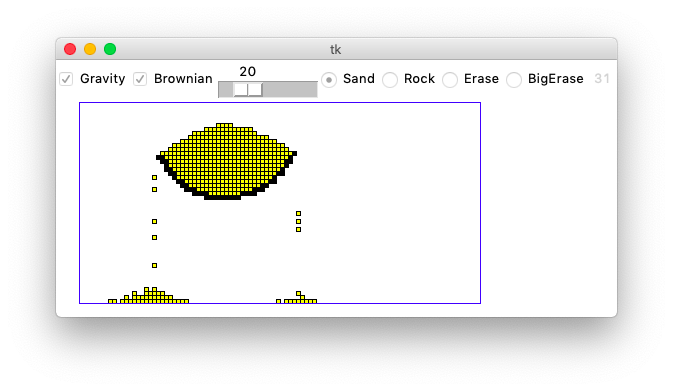The Sand program is pretty demanding on your computer. It runs a lot of computation without pause. You may notice the fans on your laptop spinning up. At the upper right of the window is a little gray number, which is the frames-per-second (fps) the program is achieving at that moment, like 31 or 52. The animation has a more fluid look with fps of, say, 50 or higher.

The more grid squares there are, and the more grains of sand there are, the slower the program runs. For each gravity round, your code needs to at least glance at every square and every grain of sand. By making the world large or by adding lots of sand, the fps of the game should go down. Play around with different grid and pixel sizes to try out the different esthetics.

## And You're Done

Once you get that all working, congratulations. That's a real program with complex logic and some neat output.

On this project we provided the """Pydoc""" sentences so you don't need to write those. When your code is working and has good cleaned up style, please turn in your waterfall.py and sand.py on paperless as usual. (Paperless link will be working Monday).

Acknowledgements: This assignment is based on the "Falling Sand" assignment by Dave Feinberg at the Stanford Nifty Assignment archive. Nick Parlante re-built it in Python, adding in the emphasis on 2-d testing and decomposition.